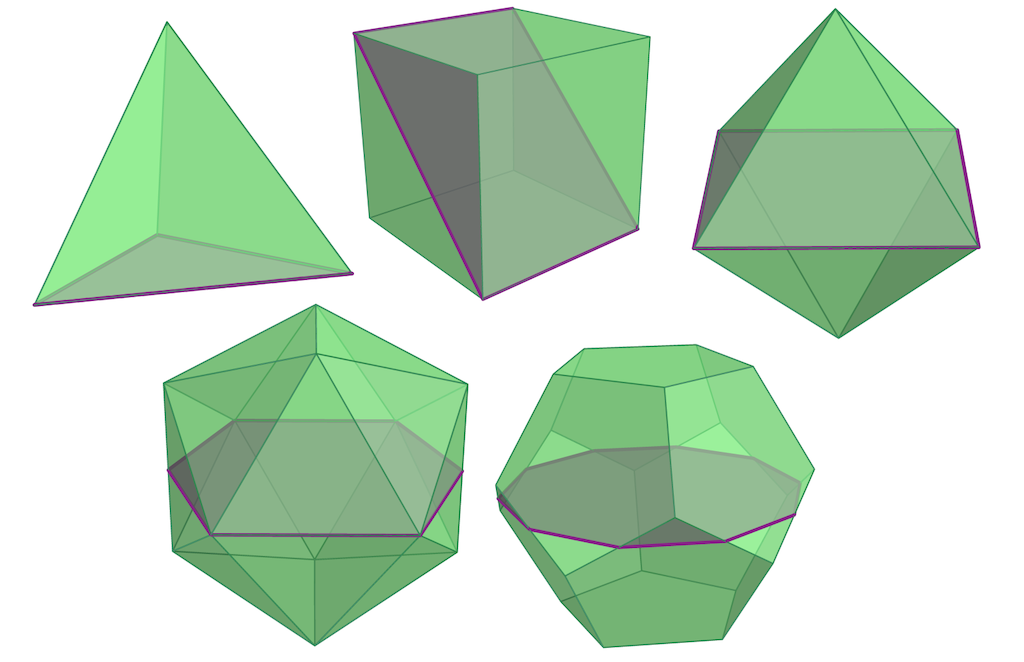### Highlights#### Slices of Polytopes

ICERM - May 2023Given a 3-dimensional cube, the intersection with an affine hyperplane is always a polygon with 3,4,5, or 6 vertices. But how can one understand the slices of a general polytope? And which slice is "the best," e.g., is the slice of maximal volume?

Sections or slices of convex bodies have been the focus of many remarkable papers in convex geometry, discrete mathematics, computational geometry, and geometric tomography. Notably, the research around the famous Busemann-Petty problem and Bourgain's slicing conjecture relates the volume of a convex body to the volume of its sections by hyperplanes [KM22]. From a discrete point of view, hyperplane sections of polytopes have shown rich combinatorial structures, and they have been studied particularly in the context of norms and specific polytopes that arise in algebraic combinatorics [B86].

In computational convexity, a fundamental question is to determine optimal or extremal hyperplane sections of convex bodies. Numerous criteria of optimality can be of interest in this context, ranging from metric criteria, such as maximizing the volume of the section, to combinatorial properties, such as maximizing the number of vertices of the section. An important related question is to determine the number of distinct combinatorial types that can arise as affine sections of a given polytope.

In the recent article “The Best Ways to Slice a Polytope” by Marie-Charlotte Brandenburg (MPI MiS), Jesús A. De Loera (UC Davis), and Chiara Meroni (ICERM), the authors study the structure of the set of all possible sections of a convex polytope by arbitrary affine hyperplanes [BLM23]. This leads to a subdivision of the space of hyperplanes into polyhedral cells with the property that all hyperplane sections coming from the same cell form a class of equivalent polytopes. Computing all such classes of hyperplanes allows for the identification of the optimal section according to numerous possible combinatorial criteria.

In each of these polyhedral cells, the volume of the hyperplane section can be expressed as a rational function, a quotient of two polynomials, in the parameters identifying the hyperplane. Finding the section of maximum volume thus turns into a global optimization problem over all polyhedral cells. With analogous strategies, one can extend these results further from hyperplane sections to orthogonal projections onto hyperplanes, and intersections with affine half-spaces.

Using the algorithms developed in the article, it is possible to find the hyperplane sections with maximal volume for any convex polytope. This is showcased for all Platonic solids, as illustrated in the picture. Another new experimental result provided by the authors is the list of all combinatorial types of slices of the cross-polytope, up to dimension 5.Platonic solidsThis work was developed at ICERM during the Spring 2023 Semester Program Discrete Optimization: Mathematics, Algorithms, and Computation.

References
[B86] Keith Ball, “Cube slicing in Rⁿ ”. In: Proceedings of the American Mathematical Society, 97:3, 1986, pp. 465–473, DOI.
[KM22] Bo’az Klartag, Vitali Milman, “The Slicing Problem by Bourgain”. In: Analysis at Large: Dedicated to the Life and Work of Jean Bourgain. Ed. by Artur Avila, Michael Th. Rassias, and Yakov Sinai. Cham: Springer International Publishing, 2022, pp. 203–231, DOI.
[BLM23] Marie-Charlotte Brandenburg, Jesús A. De Loera, Chiara Meroni, “The Best Ways to Slice a Polytope,” 2023, arXiv:2304.14239.